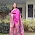## Tuesday, January 27, 2015

### Angular Dispersion and Dispersive Power

Dispersion of light

The phenomena of white light splitting up into different colors when passed through the prism is called dispersion of light. Dispersion happens because refractive index of prism is different for different wavelengths. The white light that strikes the prism surface has multiple colors with different wavelengths. As each wavelength has different refractive index, each color deviates differently trained generates group of colors. This phenomenon of splitting up of white light into multiple colors when it is passing through a material like prism is called as dispersion.

The deviation of the light ray from the original path depends on the refractive index of the material for a given color. We know that among all the colors readies having a highest wavelength and hence lowest refractive index. As a result among all the colors red deviates least and the violet deviates most.

The deviation and refractive index of a local is considered as mean value because it is approximately the average of all the visible colors.

Angular dispersion

The difference in the angle of deviation of any pair of colors is called angle disposition for these two colors.

Angle of deviation is highest for violet color and the lowest for red color. The general angular dispersion that is taken into consideration is the difference between angle of deviation of violet color and the red color.

Angular dispersion depends on the nature of the material of the prism and the angle of the prism.

Dispersive power

The ability of a prism material to disperse the light rays is measured with dispersive power.

It is defined as the ratio of angular disposition between red and violet colors to the main deviation of the colors. As the yellow color deviation is close to the average value, it is treated as the average deviation value and the average refractive index value.

Dispersive power is independent of angle of incidence but depends on the nature of the material.

Dispersion is the phenomena of splitting up of white light into multiple colors. Deviation is the phenomena of taking a different path from the original path after passing through the prism. It is very clear from the discussion that when the light ray passes through a prism it experience both the dispersion as well as the deviation.

Because of the dispersion we are able to see multiple images of a single object which takes the clarity away. Because of the deviation also the original of the image is lost. Though we cannot contain both of them, at least we can eliminate one of them.

We can calculate the total deviation as well as the total desperation generated by the combination of two different prisms as shown below.

Deviation without dispersion

We can use the combination of two different prisms so that they together can produce only deviation and there will not be any dispersion. There is no splitting up of the white color into multiple colors. The phenomena of the white light splitting into multiple colours and creating aberration in the image are called chromatic aberration. With this combination we can eliminate the chromatic aberration and hence the combination is called achromatic combination.

The condition for the deviation without for the dispersion using to prisms is derived as shown below.

Dispersion without deviation

We can arrange the combination of the two prisms in such a way that they will produce only dispersion but not any deviation. This way of arrangement is also called direct vision prism. It can be notice that the emergent light ray from the combination of the two prisms is parallel to the incident light Ray. And hence there is no deviation and there is only dispersion existing. We can derive the equation further as shown below.

Related Posts

1.2.